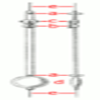#### You may also like### The Not-so-simple Pendulum 1

See how the motion of the simple pendulum is not-so-simple after all.### The Not-so-simple Pendulum 2

Things are roughened up and friction is now added to the approximate simple pendulum• The period could be expressed as $T = \alpha \times m^{A}g^{B}l^{C}$ where we need to choose A, B, C such that the dimensions on both sides of equation agrees. $\alpha$ is a constant which can be found by experiment.
• The acceleration of gravity has dimensions $L/T^2$ (meter per second squared).